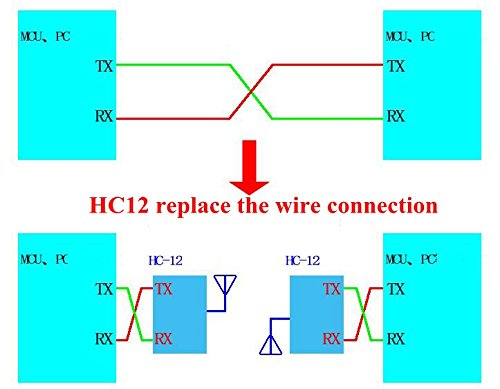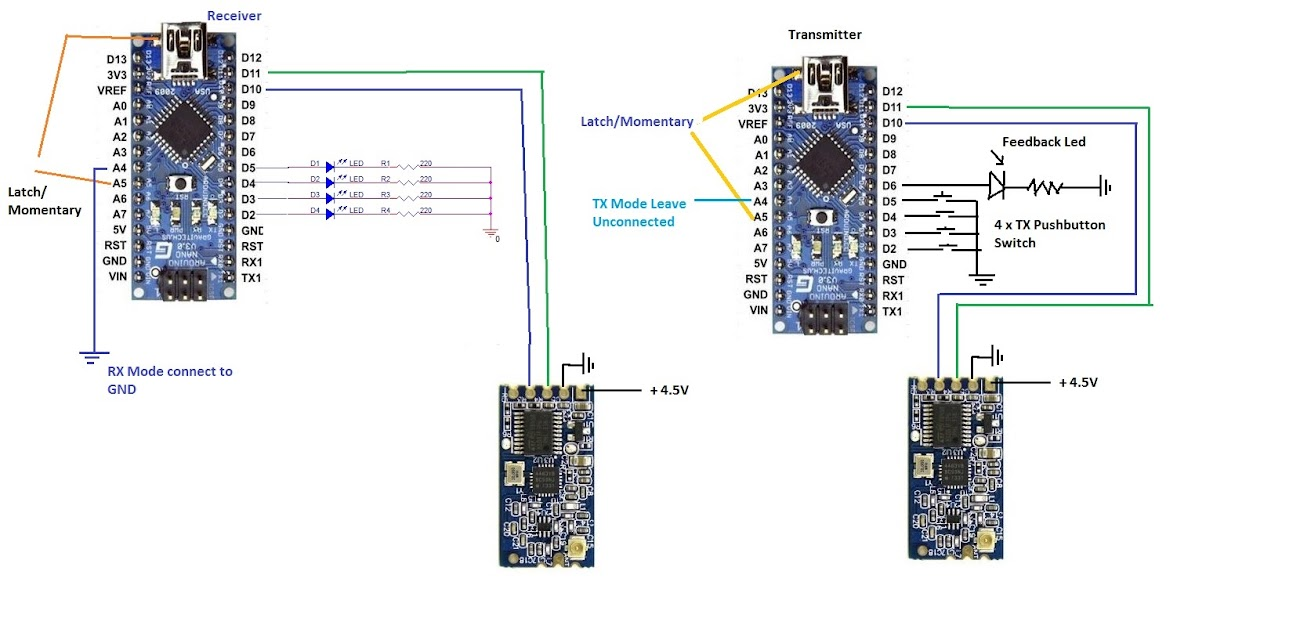# Wireless HC12 SI4463 Serial Port Transceiver Module

• 58.00 AED
• Ex Tax:58.00 AED
• Product Code: Wireless RF HC12 SI4463
• Availability: In Stock
HC12 HC-12 SI4463 Wireless Serial Port Module is a multichannel embedded wireless transceiver module with 100 communication channel, long transmission distance up to 1000m in the open air.used in Arduino wireless project or any MCU with UART (Serial Connections), Stamp holes edge design support SMD..

HC12 HC-12 SI4463 Wireless Serial Port Module is a multichannel embedded wireless transceiver module with 100 communication channel, long transmission distance up to 1000m in the open air.used in Arduino wireless project or any MCU with UART (Serial Connections), Stamp holes edge design support SMD installing easy to embed into the application devices. On-board SMA(ANT1) and solder joint(ANT2)two type antenna connecting port, you can install the SMD coaxial antenna, helical antenna or DIY antenna perfect for DIY short distance wireless data transmission system such as a wireless sensor, smart building safeguard system, automation data acquisition, etc

#### Features

Long-distance wireless transmission (1,000m in open space/baud rate 5,000bps in the air)
Working channels ( up to 100 communication channels)
Maximum 100mW (20dBm) transmitting power (8 gears of power can be set)
Three working modes, adapting to different application situations
Built-in MCU, performing communication with an external device through a serial port

Specification

Supply voltage: 3.2V to 5.5VDC
Communication distance: 1,000m in the open space
Serial baud rate: 1.2Kbps to 115.2Kbps（default 9.6Kbps）

• Receiving sensitivity: -117dBm to -100dBm
• Transmit power adjustable: -1dBm to 20dBm (default maximum)
• Interface protocol: UART/TTL
• Operating temperature: -40℃ to +85℃
• Dimensions: 27.8mm x 14.4mm x 4mm

Application

• Sensor Networks / Data collection
• Wireless metering
• Access control / Identity discrimination
• IT home appliance
• Smart house products / Security Systems
• Remote control / Remote measurement system
• Weather stations

Typical Application

When setting an RF serial data communication between microcontrollers or a microcontroller to PC, the RF modem is most useful and easy to implement.Pin definition

• VCC = Regulated 3.3V or 5V supply input of at least 200mA current capacity.
• GND = Ground level of power supply. It must be a common ground with a microcontroller.
• RDX = Receive Input: Input serial data of 3 to 5V logic level, Usually connected to TXD pin of microcontrollers UART
• TXD = Transmit Output: Output serial data of 3 to 5V logic level, Usually connected to RDX pin of microcontrollers UART
• SET = Setting Mode (Internally Pulled High) - Leave floating(High) is the normal mode. To enter config mode pull the pin low either from a switch or external MCU and send AT commands to configure.Arduino Connections with the Wireless HC12 SI4463 Serial Port Module As sender and receiver with 2 Arduino nano:Arduino code for Wireless HC12 SI4463 Serial Port Module using the top connections with the two Arduino nano:

```/*
* This includes: Latch Mode, Momentary Mode (selected by A5 input), and also feedback to Transmitter that the Receiver got transmission OK - by LED on Transmitter
* Controls 4 logic output at 2 modes: Latch (each press change output ON/OFF), Momentary (output on just when button is pressed)
*HC-12 Moule Transciever Same code is for Transmitter and Receiver, setup through A4 Input
*Tested on Arduino Nano
* A4=1=> TX, A4=0=>RX
* A5=1=>LATCH (Default mode), A5=0=>Momentary the stting now is via variable latch_logic if 1=> latch if 0=> momentary
*
* NANO connections to HC-12 As follows:
* ARDUINO NANO HC-12-MODULE
* _________________________________________________________________________
* 5V -> 1N4148 Anode, 1N4148 catode -> HC-12/Pin-1 (VCC)
* GND -------> GND
* D11 -------> RXD
* D10 -------> TXD
*
* NANO (TX MODULE) NANO (RX MODULE)
* ______________________ ________________________________
* D2 --> SW --> GND (SW1) A4 --> GND
* D3 --> SW --> GND (SW2) D2 --> OUTPUT 1
* D4 --> SW --> GND (SW3) D3 --> OUTPUT 2
* D5 --> SW --> GND (SW4) D4 --> OUTPUT 3
* D5 --> OUTPUT 4
*
* FEEDBACK back will light Led on Trnasmitter for 100mS

*
*/
#define P1 2
#define P2 3
#define P3 4
#define P4 5
#define F1 6 // feedback back to transmitter
//#define latch_logic 1 // if 1 then latch if 0 momentary
#include
SoftwareSerial mySerial(10, 11); // RX, TX
char latch; // , if latch=1 then Latch
int TX_CODE, RX_READ, mode, TX_CODE_OLD; //if mode= 1 then TX
int RP1, RP2, RP3, RP4;
volatile byte SW1 = 0, SW2 = 0, SW3 = 0, SW4 = 0;
boolean S1, S2, S3, S4;
byte TEST;
char TXTOSEND,feedback;
int FLAG=1,latch_logic;
void setup() {
Serial.begin(9600);
mySerial.begin(9600);
Serial.println("Hello HC-12 TX and RX SW, A4-Low=TX A4-HIGH=RX A5-LOW=Momemtary A5-HIGH=LATCH");
// mySerial.println("Hello HC-12 TX and RX SW, A4-Low=TX A4-HIGH=RX A5-LOW=Momemtary A5-HIGH=LATCH");
// This is for internal pull ups resistors on A4 and A5 Inputs
delay(500);
pinMode(A4, INPUT_PULLUP);
pinMode(A5, INPUT_PULLUP);
mode = 1;
Serial.println("TX Mode");
}
else
{
mode = 0;
Serial.println("RX Mode");
}
latch = 1;
latch_logic=latch;
Serial.println("LATCH Mode");
}
else
{
latch = 0;
latch_logic=latch;
Serial.println("Momentary Mode");
}
if (mode == 1) // TX
{
pinMode(P1, INPUT); // set pin to input
digitalWrite(P1, HIGH); // turn on pullup resistors
pinMode(P2, INPUT); // set pin to input
digitalWrite(P2, HIGH); // turn on pullup resistors
pinMode(P3, INPUT); // set pin to input
digitalWrite(P3, HIGH); // turn on pullup resistors
pinMode(P4, INPUT); // set pin to input
digitalWrite(P4, HIGH); // turn on pullup resistors
pinMode(F1, OUTPUT); // set pin to input
digitalWrite(F1, LOW);
}
else
{
pinMode(P1, OUTPUT); // set pin to input
digitalWrite(P1, LOW); // turn on pullup resistors
pinMode(P2, OUTPUT); // set pin to input
digitalWrite(P2, LOW); // turn on pullup resistors
pinMode(P3, OUTPUT); // set pin to input
digitalWrite(P3, LOW); // turn on pullup resistors
pinMode(P4, OUTPUT); // set pin to input
digitalWrite(P4, LOW); // turn on pullup resistors
}
}
void loop() {
if (mode == 1) //TX Mode
{
TX_MODE();
}
else
{
RX_MODE();
}
}
void TX_MODE()
{
while (1)
{
{
TX_CODE = 64 + (read_P1 * 1) + (read_P2 * 2) + (read_P3 * 4) + (read_P4 * 8); // converting TX to one char
TXTOSEND = TX_CODE; // conver to char for sending OK
if (FLAG==0) // skip first time on FLAG=1
{
if (latch==1)
{
if ((TX_CODE != TX_CODE_OLD)&&(latch_logic==1)) // transmit only on change
{
Serial.print(TX_CODE, BIN);
mySerial.print(TXTOSEND);
}
delay(100);
TX_CODE_OLD = TX_CODE;
}
else
{
Serial.print(TX_CODE, BIN);
mySerial.print(TXTOSEND);
delay(100);
}
}
else FLAG=0;
}
if (feedback=='R')
{
Serial.println("Data_Sent_OK"); // feedback to serial port
digitalWrite(F1, HIGH); // Ligjt Led Back on Transmitter
delay(80);
digitalWrite(F1, LOW);
}
}
}
void RX_MODE()
{
while (1)
{
if (mySerial.available()) {
RP1 = (TEST) & (1);
RP2 = (TEST) & (2);
RP3 = (TEST) & (4);
RP4 = (TEST) & (8);
mySerial.flush();
// When button is pressed (logic 0) toggling the apropriate LED Latch mode only
if ((latch==1)&&(latch_logic==1))
{
if (RP1 == 0) SW1 = ~(SW1);
if (RP2 == 0) SW2 = ~(SW2);
if (RP3 == 0) SW3 = ~(SW3);
if (RP4 == 0) SW4 = ~(SW4);
mySerial.print("R"); // This is feedback back to transmitter
}
else // Momentary mode ON only when button is pressed continously
{
if (RP1 == 0)
{
SW1 = 1;
mySerial.print("R"); // This is feedback back to transmitter
} else SW1=0;
if (RP2 == 0)
{
SW2 = 1;
mySerial.print("R"); // This is feedback back to transmitter
} else SW2=0;
if (RP3 == 0)
{
SW3 = 1;
mySerial.print("R"); // This is feedback back to transmitter
} else SW3=0;
if (RP4 == 0)
{
SW4 = 1;
mySerial.print("R"); // This is feedback back to transmitter
} else SW4=0;
}
//convert to bit for Digital-Out from Arduino
S1= SW1 & 1;
S2= SW2 & 1;
S3= SW3 & 1;
S4= SW4 & 1;
digitalWrite(P1, S1);
digitalWrite(P2, S2);
digitalWrite(P3, S3);
digitalWrite(P4, S4);
delay(100);
Serial.print("S1=");
Serial.print(SW1);
Serial.print(" S2=");
Serial.print(SW2);
Serial.print(" S3=");
Serial.print(SW3);
Serial.print(" S4=");
Serial.println(SW4);
}
}

}```

There are no reviews for this product.